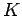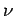# 2.5: Photoelectric Effect

$$\newcommand{\vecs}{\overset { \rightharpoonup} {\mathbf{#1}} }$$ $$\newcommand{\vecd}{\overset{-\!-\!\rightharpoonup}{\vphantom{a}\smash {#1}}}$$$$\newcommand{\id}{\mathrm{id}}$$ $$\newcommand{\Span}{\mathrm{span}}$$ $$\newcommand{\kernel}{\mathrm{null}\,}$$ $$\newcommand{\range}{\mathrm{range}\,}$$ $$\newcommand{\RealPart}{\mathrm{Re}}$$ $$\newcommand{\ImaginaryPart}{\mathrm{Im}}$$ $$\newcommand{\Argument}{\mathrm{Arg}}$$ $$\newcommand{\norm}{\| #1 \|}$$ $$\newcommand{\inner}{\langle #1, #2 \rangle}$$ $$\newcommand{\Span}{\mathrm{span}}$$ $$\newcommand{\id}{\mathrm{id}}$$ $$\newcommand{\Span}{\mathrm{span}}$$ $$\newcommand{\kernel}{\mathrm{null}\,}$$ $$\newcommand{\range}{\mathrm{range}\,}$$ $$\newcommand{\RealPart}{\mathrm{Re}}$$ $$\newcommand{\ImaginaryPart}{\mathrm{Im}}$$ $$\newcommand{\Argument}{\mathrm{Arg}}$$ $$\newcommand{\norm}{\| #1 \|}$$ $$\newcommand{\inner}{\langle #1, #2 \rangle}$$ $$\newcommand{\Span}{\mathrm{span}}$$$$\newcommand{\AA}{\unicode[.8,0]{x212B}}$$

The so-called photoelectric effect, by which a polished metal surface emits electrons when illuminated by visible and ultra-violet light, was discovered by Heinrich Hertz in 1887 . The following facts regarding this effect can be established via careful observation. First, a given surface only emits electrons when the frequency of the light with which it is illuminated exceeds a certain threshold value, which is a property of the metal. Second, the current of photoelectrons, when it exists, is proportional to the intensity of the light falling on the surface. Third, the energy of the photoelectrons is independent of the light intensity, but varies linearly with the light frequency. These facts are inexplicable within the framework of classical physics.

In 1905, Albert Einstein proposed a radical new theory of light in order to account for the photoelectric effect . According to this theory, light of fixed frequency $$\nu$$ consists of a collection of indivisible discrete packages, called quanta,1 whose energy is

$\label{ee3.15} E = h\,\nu.$ Here, $$h = 6.6261\times 10^{-34}\,{\rm J\,s}$$ is a new constant of nature, known as Planck’s constant. Incidentally, $$h$$ is called Planck’s constant, rather than Einstein’s constant, because Max Planck first introduced the concept of the quantization of light, in 1900, while trying to account for the electromagnetic spectrum of a black body (i.e., a perfect emitter and absorber of electromagnetic radiation) .

Suppose that the electrons at the surface of a metal lie in a potential well of depth $$W$$. In other words, the electrons have to acquire an energy $$W$$ in order to be emitted from the surface. Here, $$W$$ is generally called the work function of the surface, and is a property of the metal. Suppose that an electron absorbs a single quantum of light. Its energy therefore increases by $$h\,\nu$$. If $$h\,\nu$$ is greater than $$W$$ then the electron is emitted from the surface with residual kinetic energy $K = h\,\nu - W.$ Otherwise, the electron remains trapped in the potential well, and is not emitted. Here, we are assuming that the probability of an electron simultaneously absorbing two or more light quanta is negligibly small compared to the probability of it absorbing a single light quantum (as is, indeed, the case for sufficiently low-intensity illumination). Incidentally, we can calculate Planck’s constant, and the work function of the metal, by simply plotting the kinetic energy of the emitted photoelectrons as a function of the wave frequency, as shown in Figure [f1]. This plot is a straight-line whose slope is $$h$$, and whose intercept with the $$\nu$$-axis is $$W/h$$. Finally, the number of emitted electrons increases with the intensity of the light because the more intense the light, the larger the flux of light quanta onto the surface. Thus, Einstein’s quantum theory is capable of accounting for all three of the previously mentioned observational facts regarding the photoelectric effect.Figure 4: Variation of the kinetic energyof photoelectrons with the wave-frequency.

$$\newcommand {\ltapp} {\stackrel {_{\normalsize<}}{_{\normalsize \sim}}}$$ $$\newcommand {\gtapp} {\stackrel {_{\normalsize>}}{_{\normalsize \sim}}}$$ $$\newcommand {\btau}{\mbox{\boldmath\tau}}$$ $$\newcommand {\bmu}{\mbox{\boldmath\mu}}$$ $$\newcommand {\bsigma}{\mbox{\boldmath\sigma}}$$ $$\newcommand {\bOmega}{\mbox{\boldmath\Omega}}$$ $$\newcommand {\bomega}{\mbox{\boldmath\omega}}$$ $$\newcommand {\bepsilon}{\mbox{\boldmath\epsilon}}$$